# Quick Sort

Updated:

⚠️ This post was created when I was in high school and is no longer maintained.

### If we say that main idea of Merge sort is recursively merge, then the quick sort is just recursively partitioning.

                                           ___ MIT

## 1. Intuition

Let A be the array we want to sort. Firstly, we partition A into 3 sets:

• Pivot set: A singleton set that only contains the pivot; (the pivot is usually the last element in the current array portion A[r])
• Greater set: Contains the elements from A[i+1] to A[j - 1] which are all greater than the pivot;
• Less & Equal set: Contain the elements from A[p] to A[i] which are all less and equal to the pivot.

### $Loop invariant (Three conditions):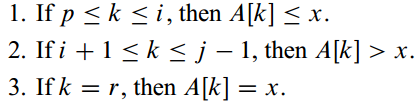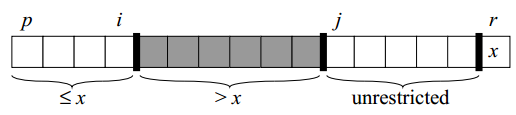###$ Maintenance: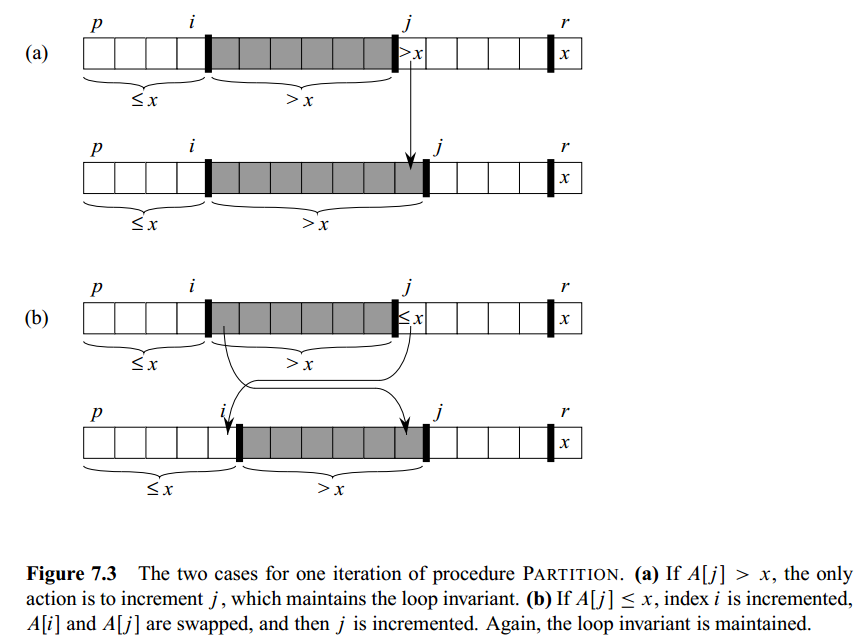## 2. Pseudocode

-- choose the last item as the pivot --
algorithm QuikSort(array, start, end):
if start < end then
pivot_i = Partition(array, start, end)

QuikSort(array, start, pivot_i-1)
QuilSort(array, pivot_i+1, end)

function Partition(array, start, end):
pivot = array[end]
i = start - 1

For j := start to (end-1) do
if array[j] ≤ pivot then
i = i + 1 --> array[i] is included in the smaller-set so we need to increase it before the exchange
Exchange array[i] with array[j]

Exchange array[i+1] with array[end]

return i+1


## 3. Time Complexity

### The running time of quicksort depends on whether the partitioning is balanced or unbalanced, which in turn depends on which elements are used for partitioning.

• Balanced partitioning :≈ merge sort
• Unbalanced partitioning :≈ insertion sort

### (1) Unbalanced Partitioning (Worst case):

The worst-case behaviour for quicksort occurs when the partitioning routine produces one subproblem with n 1 elements and one with 0 elements.

$T(n) = T(n-1) + T(0) + \Theta(n) \\ = T(n-1) + \Theta(n)$ Then, we can use subsitution method to prove that: $T(n) = T(n-1)+\Theta(n) \\ = \Theta(n^2)$

### (2) Best Partitioning (Best case):

In the most even possible split, PARTITION produces two subproblems, each of size no more than n=2, since one is of size floor(n/2) and one of size (ceil(n/2)-1). In this case, quicksort runs much faster. $T(n) = 2T(n/2) + \Theta(n)$ By case 2 of the master theorem (Theorem 4.1), this recurrence has the solution: $T(n) = \Theta(nlgn)$

### (3) Balanced Partitioning (Average case)

Any split of constant proportionality yields a recursion tree of depth Θ(lgn) where the cost at each level is O(n)

The running time is, therefore, O(nlgn) whenever the split has constant proportionality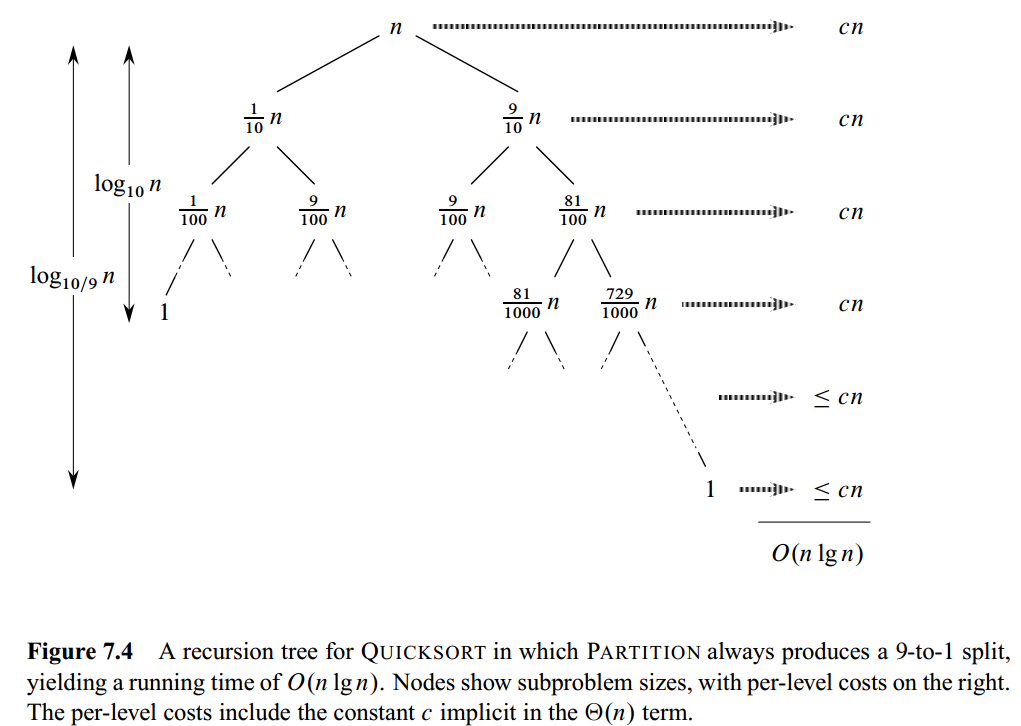#### Tips: Intuition for average-case

• For a random input array, the partitioning is different at every level;
• The average-case ≈ a mix of “good” and “bad” partitioning (good: constant proportionality; bad: unbalanced);
• The “good” and “bad” splits are distributed randomly through the tree.
• The depth of the tree is always O(lgn) and O(n) work is performed at each level.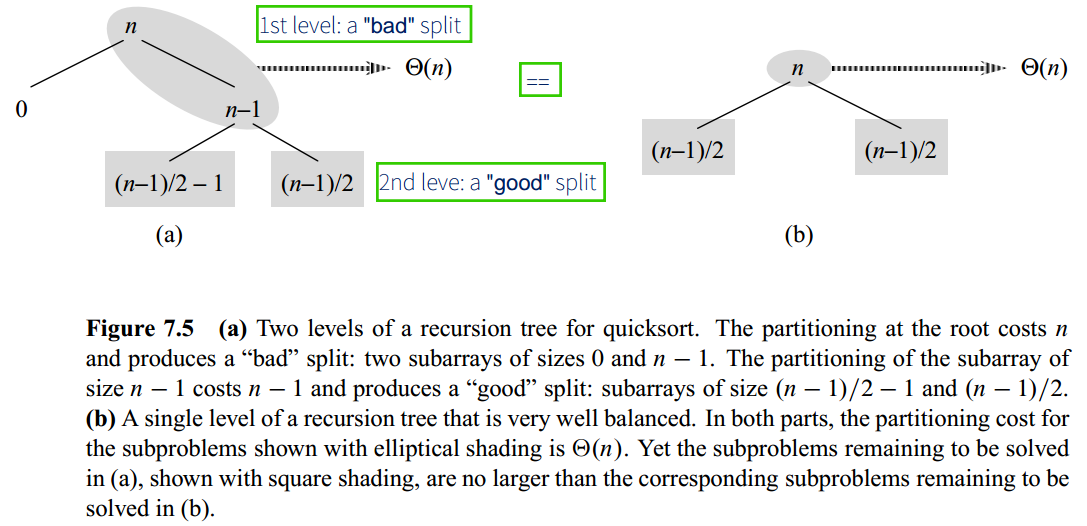(bad+good ≈ good)

## Code

// Scala
object QuickSortAlgo {

def quickSort(array: Array[Double], start: Int, end: Int): Unit = {
if (start < end) {
var pivot_i = partition(array, start, end)

quickSort(array, start, pivot_i-1)
quickSort(array, pivot_i+1, end)
}
}

def partition(array: Array[Double], start: Int, end: Int): Int = {
var pivot = array(end)
var i = start - 1

for (j <- start until end) {
if (array(j) < pivot) {
i += 1
// swap
var tmp = array(i)
array(i) = array(j)
array(j) = tmp
}
}
var tmp = array(i+1)
array(i+1) = array(end)
array(end) = tmp

return i+1
}

def main(args: Array[String]): Unit = {
var array = Array(4234,234234,23,234,-134,4123,41,578,-342,55,0.2323,0.325)
println("\nBefore sorting:")
println(array.deep.mkString(" "))

quickSort(array, 0, array.length-1)

println("\nAfter sorting:")
println(array.deep.mkString(" "))
}
}

# Python
def partition(array, start, end):
pivot = array[end]
i = start - 1

for j in range(start, end):
if array[j] <= pivot:
i += 1
array[i], array[j] = array[j], array[i]

array[i+1], array[end] = array[end], array[i+1]

return i+1

def quick_sort(array, start, end):
if start < end:
pivot_i = partition(array, start, end)

quick_sort(array, start, pivot_i-1)
quick_sort(array, pivot_i+1, end)

if __name__ == "__main__":
array = [4234,234234,23,234,-134,123,41,578,-342,55,0.9323,0.325]
# array = [10, 9, 8, 7, 6, 5, 4, 3, 2, 1, 0]

print("Before sorting: ", array)
quick_sort(array, 0, len(array)-1)
print("After sorting:  ", array)



Tags: